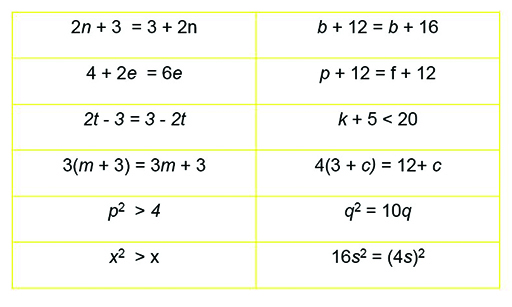Teaching mathematics

Start this free course now. Just create an account and sign in. Enrol and complete the course for a free statement of participation or digital badge if available.

Free course

# 3.2 Always, sometimes, never true?

As previously discussed, algebraic expressions are like mathematical phrases or sentences. Equations are like mathematical statements. A mathematician will always ask whether or not an equation is true.

When equations contain no unknown quantities, use calculation to check if they are true:

is an equation but it is not true.

is an equation that is true.

When equations include unknown quantities, they may be true for only one value of the unknown:

is only true when n is 11.

If n = 10 then 2n – 5 = 17 is false.

They may be true for several values:

is true when n = 3 and when n = –3.

They may be true for all values of the unknown:

In formal mathematics you can use the sign ≡ to show that these expressions are ‘identically equal’:

Sometimes an equation is given as a definition or a formula for working something out. We can assume that someone else has done the work of showing it is always true. For example:

(definition).

(formula a learner could prove).

(formula that a learner could not prove!).

## Activity 15 Always, sometimes or never true

Timing: Allow 10 minutes

Decide whether these equations are true for all values of the variable, or only some values or none.Figure 9 Always, sometimes, never true?

### Discussion

Trying values will help you see that there are no values of b or m that make their equations true: they are never true. Using algebraic reasoning will help us see that e = 1, t = 1.5, c = 0, q = 10 are specific values that make their respective equations true. Also, some equations are true for a range of values: k < 15, p either greater than 2 or less than -2, and x greater than 1 or less than 0. A final one, that is more subtle because it involves two variables, is that p + 12 = f + 12 is true only when p = f. All these equations are sometimes true.

Finally the equations 2n+3 = 3+2n and 16s2 = (4s)2 are true for all values of n and s. The first is because addition is commutative, and the second because squaring applies to the whole bracket. They are always true.

TM_1